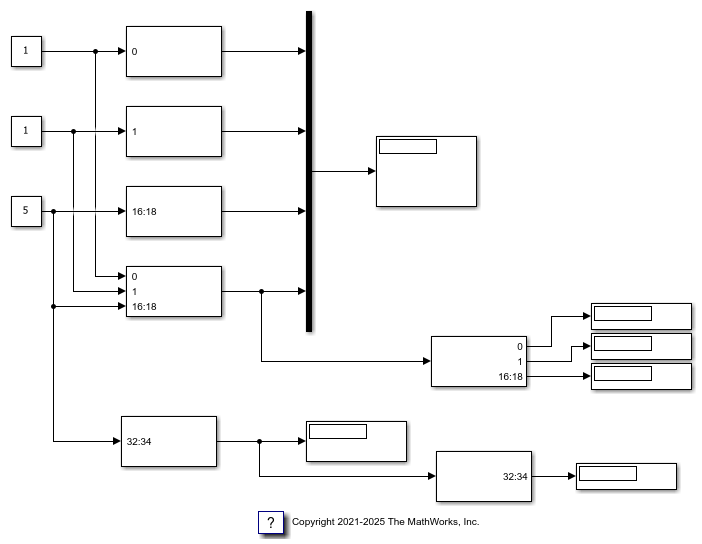# Configure Input and Output Ports for Bit Packing and Unpacking

This example shows how to configure Bit Packing blocks and Bit Unpacking blocks.

### Open Model

The model uses Bit Packing, Bit Unpacking, and Display blocks to show how to use the input and output port configuration syntax. Open the model and double-click blocks to view the configuration.

```model = 'slrt_ex_bit_pack_unpack'; open_system(fullfile(matlabroot,'toolbox','slrealtime','examples',model)); ```### Settings for Bit Packing and Bit Unpacking Blocks

To demonstrate the syntax for configuring block input and output ports, the model `slrt_ex_bit_pack_unpack` has a set of Bit Packing blocks and Bit Unpacking blocks that use these settings:

slrt_ex_bit_pack_unpack/Bit Packing

• Bit patterns: `{}`

• Output port (packed) data type: `uint32`

• Outpupt port (packed) dimensions: ``

slrt_ex_bit_pack_unpack/Bit Packing1

• Bit patterns: `{}`

• Output port (packed) data type: `uint32`

• Outpupt port (packed) dimensions: ``

slrt_ex_bit_pack_unpack/Bit Packing2

• Bit patterns: `{[16:18]}`

• Output port (packed) data type: `uint32`

• Outpupt port (packed) dimensions: ``

slrt_ex_bit_pack_unpack/Bit Packing3

• Bit patterns: `{0, 1, [16:18]}`

• Output port (packed) data type: `uint32`

• Outpupt port (packed) dimensions: ``

slrt_ex_bit_pack_unpack/Bit Packing4

• Bit patterns: `{[32:34]}`

• Output port (packed) data type: `uint32`

• Outpupt port (packed) dimensions: ``

slrt_ex_bit_pack_unpack/Bit Unpacking

• Bit patterns: `{0, 1, [16:18]}`

• Input port (packed) data type: `uint32`

• Inpupt port (packed) dimensions: ``

• Ouptut port (unpacked) data types (cell array): `{'uint8','uint16', 'uint32'}`

• Output port (unpacked) dimensions (cell array): `{1,1,1}`

• Sign extended: 'On'

slrt_ex_bit_pack_unpack/Bit Unacking1

• Bit patterns: `{[32:34]}`

• Input port (packed) data type: `uint32`

• Inpupt port (packed) dimensions: ``

• Ouptut port (unpacked) data types (cell array): `{'uint8'}`

• Output port (unpacked) dimensions (cell array): `{1}`

• Sign extended: 'On'

### Build Model and Run Real-Time Application

To show the unpacked output in the Display blocks, build the model and run the real-time application.

```evalc('slbuild(model)'); tg = slrealtime; load(tg,model); start(tg); pause(10); stop(tg); ```

### Close Model

```bdclose('all'); ```